• 机器人速度规划算法，包括7种速度规划算法：梯形、余弦、多项式、7段s型，7段修正s型，15段s型，31段s型速度规划。所有核心算法均用c语言实现，不调用第三方算法库。
• 梯形加减速的速度规划，可以根据起始点位置、速度，终点位置、速度，按照设定的速度和加减速度进行速度规划
• 自己写的一个简单的T型速度规划m文件。需要给定最大的加速度、运行时间、开始角度 、结束角度。
•   梯形速度规划是最快也是最简单的速度规划方法。由于其速度连续但不平滑，加速度可控但会跳变，加加速度不可控，通常会引起受控对象震动，控制效果较差。S形速度规划由于其速度连续且平滑，加速度可控且连续，加...


文章目录
一、问题描述二、MATLAB代码三、总结

一、问题描述
梯形速度规划是最快也是最简单的速度规划方法。由于其速度连续但不平滑，加速度可控但会跳变，加加速度不可控，通常会引起受控对象震动，控制效果较差。S形速度规划由于其速度连续且平滑，加速度可控且连续，加加速度可控，控制效果好，广泛用于工业现场。但是，S形速度规划算法较复杂，在某些条件下，需要迭代计算进行规划，计算时间稍长。那么，有没有这样的方法：采用简单的梯形速度规划方法得到位置曲线，再通过一些方法，生成平滑的S形位置曲线？有的！本文提供了两种方法，效果如下：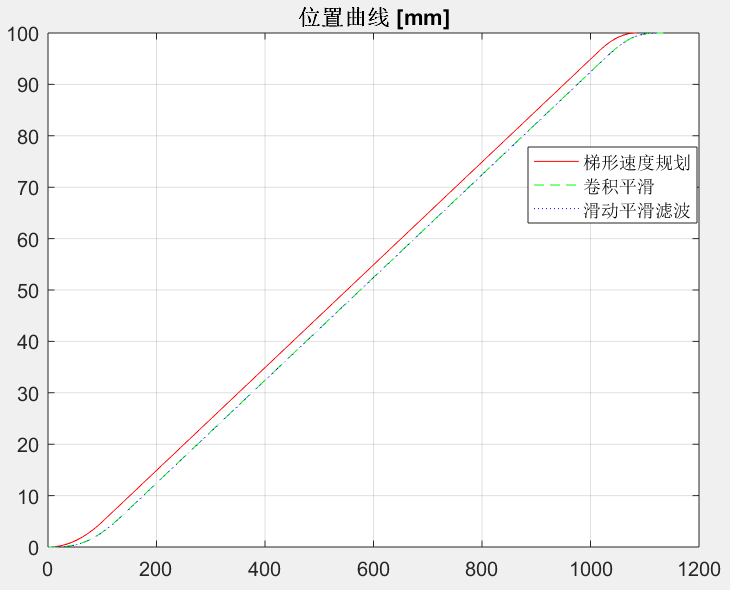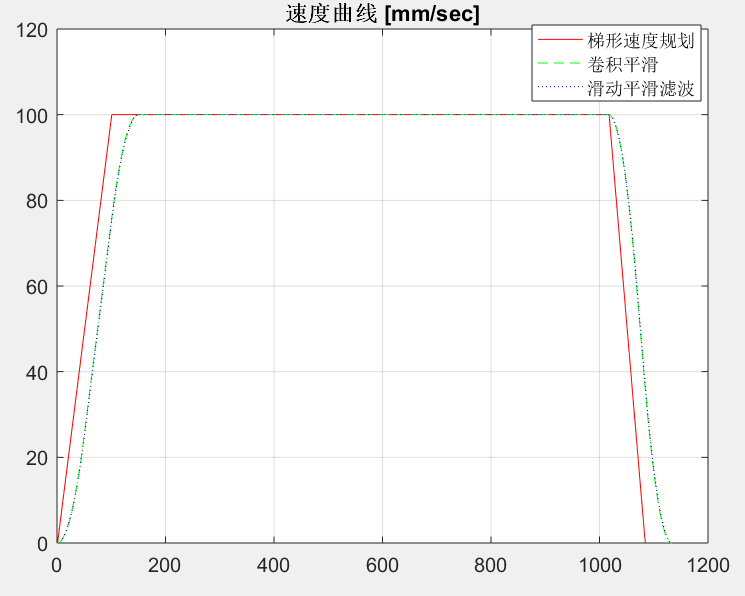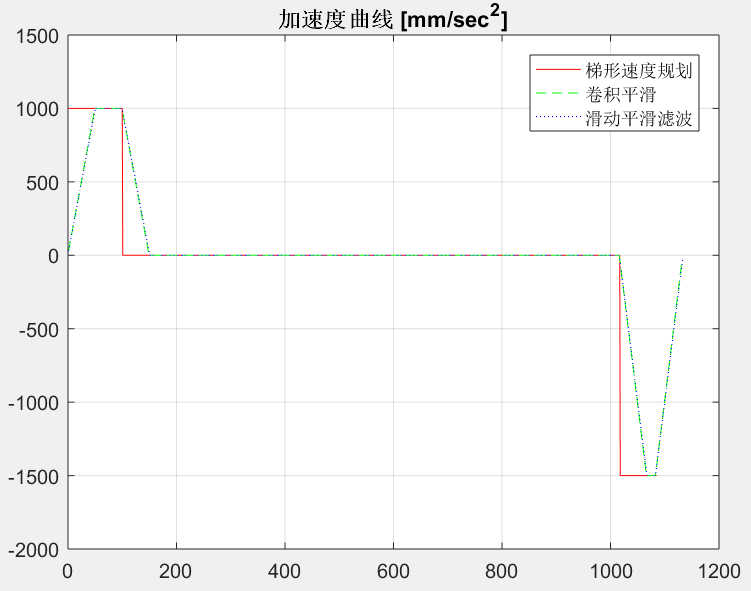二、MATLAB代码
计算两列数据的卷积：
%{
Function: my_conv
Description: 计算两列数据的卷积
Input: 数据列u，数据列v
Output: 数据列w
Author: Marc Pony(marc_pony@163.com)
Date: 2020.04.06
%}
function w = my_conv(u, v)
m = length(u);
n = length(v);
count = m + n - 1;
w = zeros(count, 1);
for k = 1 : count
w(k) = 0.0;
startIndex = max([1, k - n + 1]);
endIndex = min([k, m]);
for j = startIndex : endIndex
w(k) = w(k) + u(j) * v(k - j + 1);
end
end
end

梯形速度规划：
%{
Function: trapezoidal_velocity_planning
Description: 梯形速度规划
Input: 运动参数motionParams
Output: 规划好了的运动参数motionParams，状态变量sta(1表示成功，0表示失败)
Author: Marc Pony(marc_pony@163.com)
Date: 2020.04.06
%}
function [motionParams, sta] = trapezoidal_velocity_planning(motionParams)
sta = 1;
if (motionParams.vs < motionParams.ve)
if (motionParams.L + eps < (motionParams.ve * motionParams.ve - motionParams.vs * motionParams.vs) / (2.0 * motionParams.acc))
sta = 0;
return;
end
elseif (motionParams.vs > motionParams.ve)
if (motionParams.L + eps < (motionParams.vs * motionParams.vs - motionParams.ve * motionParams.ve) / (2.0 * motionParams.dec))
sta = 0;
return;
end
else
%to do nothing
end

motionParams.vm = sqrt((2.0 * motionParams.acc * motionParams.dec * motionParams.L + motionParams.dec * motionParams.vs * motionParams.vs + motionParams.acc * motionParams.ve * motionParams.ve) / (motionParams.acc + motionParams.dec));
if (motionParams.vm > motionParams.vc)
motionParams.vm = motionParams.vc;
end

motionParams.T1 = (motionParams.vm - motionParams.vs) / motionParams.acc;
motionParams.T3 = (motionParams.vm - motionParams.ve) / motionParams.dec;

motionParams.L1 = 0.5 * (motionParams.vm + motionParams.vs) * motionParams.T1;
motionParams.L3 = 0.5 * (motionParams.vm + motionParams.ve) * motionParams.T3;
motionParams.L2 = motionParams.L - motionParams.L1 - motionParams.L3;
motionParams.T2 = motionParams.L2 / motionParams.vm;

motionParams.t1 = motionParams.T1;
motionParams.t2 = motionParams.t1 + motionParams.T2;
motionParams.t3 = motionParams.t2 + motionParams.T3;
motionParams.totalT = motionParams.t3;

motionParams.s1 = motionParams.L1;
motionParams.s2 = motionParams.s1 + motionParams.L2;
motionParams.s3 = motionParams.s2 + motionParams.L3;
end

梯形速度规划的主轴插补：
%{
Function: main_axis_interpolation_for_trapezoidal_velocity
Description: 梯形速度规划的主轴插补
Input: 已经规划好了的运动参数motionParams
Output: 主轴位置pos(mm)、主轴速度vel(mm/s)、主轴加速度acc(mm/s^2)
Author: Marc Pony(marc_pony@163.com)
Date: 2020.04.06
%}
function [pos, vel, acc] = main_axis_interpolation_for_trapezoidal_velocity(motionParams)
if (motionParams.t < motionParams.t1)
pos = motionParams.vs * motionParams.t + 0.5 * motionParams.acc * motionParams.t * motionParams.t;
vel = motionParams.vs + motionParams.acc * motionParams.t;
acc = motionParams.acc;
elseif (motionParams.t < motionParams.t2)
pos = motionParams.L1 + (motionParams.t - motionParams.t1) * motionParams.vm;
vel = motionParams.vm;
acc = 0.0;
elseif (motionParams.t < motionParams.t3)
pos = motionParams.L1 + motionParams.L2 + motionParams.vm * (motionParams.t - motionParams.t2) - 0.5 * motionParams.dec * (motionParams.t - motionParams.t2) * (motionParams.t - motionParams.t2);
vel = motionParams.vm - motionParams.dec *(motionParams.t - motionParams.t2);
acc = -motionParams.dec;
else
motionParams.t = motionParams.totalT;
pos = motionParams.L;
vel = motionParams.ve;
acc = -motionParams.dec;
end

end

采用梯形速度规划生成S形速度曲线(卷积平滑方法和滑动平滑滤波方法)：
clc;
clear;
close all;

%% 输入参数
motionParams = struct();
motionParams.dt = 0.001;  %s
motionParams.L = 100;     %mm
motionParams.vs = 0;      %mm/s
motionParams.ve = 0;      %mm/s
motionParams.vc = 100;    %mm/s
motionParams.acc = 1000;  %mm/s^2
motionParams.dec = 1500;  %mm/s^2
smoothFactor = 50; %平滑因子，用于衡量加加速度，非负整数，时间增加smoothFactor*插补周期

%% 梯形速度规划
[motionParams, sta] = trapezoidal_velocity_planning(motionParams);
if sta == 0
error('当前运动参数下无法规划！');
end

%% 梯形速度插补
count = ceil(motionParams.totalT / motionParams.dt) + 1;
time = zeros(count, 1);
pos = zeros(count, 1);
vel = zeros(count, 1);
acc = zeros(count, 1);
vel(1) = motionParams.vs;
acc(1) = motionParams.acc;
motionParams.t = 0.0;
for i = 2 : count
motionParams.t = motionParams.t + motionParams.dt;
[pos(i), vel(i), acc(i)] = main_axis_interpolation_for_trapezoidal_velocity(motionParams);
time(i) = motionParams.t;
end

%% 方法1：卷积平滑
newPos1 = [pos ; ones(smoothFactor, 1) * pos(end)];
temp = ones(smoothFactor, 1) / smoothFactor;
smoothNewPos1 = my_conv(newPos1, temp);
smoothNewPos1 = smoothNewPos1(1 : length(newPos1));
smoothNewVel1 = diff(smoothNewPos1) / motionParams.dt;
smoothNewAcc1 = diff(smoothNewVel1) / motionParams.dt;

%% 方法2：滑动平滑滤波
newPos2 = [ones(smoothFactor, 1) * pos(1); pos; ones(smoothFactor, 1) * pos(end)];
count = length(pos) + smoothFactor;
smoothNewPos2 = zeros(count, 1);
for i = 1 : count
smoothNewPos2(i) = mean(newPos2(i : i + smoothFactor));
end
smoothNewVel2 = diff(smoothNewPos2) / motionParams.dt;
smoothNewAcc2 = diff(smoothNewVel2) / motionParams.dt;

%% 绘图验证结果
figure(1);
plot(pos, 'r');
hold on
plot(smoothNewPos1, 'g--');
plot(smoothNewPos2, 'b:');
legend('梯形速度规划', '卷积平滑', '滑动平滑滤波')
grid;
title('位置曲线 [mm]');

figure(2);
plot(vel, 'r');
hold on
plot(smoothNewVel1, 'g--');
plot(smoothNewVel2, 'b:');
legend('梯形速度规划', '卷积平滑', '滑动平滑滤波')
grid;
title('速度曲线 [mm/sec]');

figure(3);
plot(acc, 'r');
hold on
plot(smoothNewAcc1, 'g--');
plot(smoothNewAcc2, 'b:');
legend('梯形速度规划', '卷积平滑', '滑动平滑滤波')
grid;
title('加速度曲线 [mm/sec^2]');

三、总结
采用梯形速度规划，采用卷积平滑方法和滑动平滑滤波方法均可以很容易生成S形速度曲线。卷积平滑方法不适用于在线插补，滑动平滑滤波方法则适用。两种方法有个缺点是：不适用于起始速度vs和结束速度ve不为0的情况。
展开全文• 2 分段式S形曲线速度规划 已知初速度V0,末速度Ve,最大速度Vmax，路程距离s，最大加速度amax,最大jerk（加加速度）jmax,要规划位置、速度、加速度、加加速度随时间变化的曲线。具体步骤如下： 1） 假设能够达到最大...
• ## S形速度规划算法

千次阅读 2020-01-14 19:19:41
S形速度规划相对于梯形速度规划其速度曲线会更加平滑，电机运行会更加平稳。常见的S曲线包括7段式加速度曲线，这种曲线计算量大，而且规划起来困难。一种简单的方法是采用Sigmoid对称函数加减速曲线规划法。典型的...
S形速度规划相对于梯形速度规划其速度曲线会更加平滑，电机运行会更加平稳。常见的S曲线包括7段式加速度曲线，这种曲线计算量大，而且规划起来困难。一种简单的方法是采用Sigmoid对称函数加减速曲线规划法。典型的Sigmoid函数为：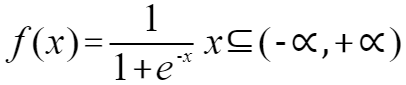其值域为(0,1)，函数关于横坐标左右对称，关于点（0,0.5）中心对称。其函数图形为：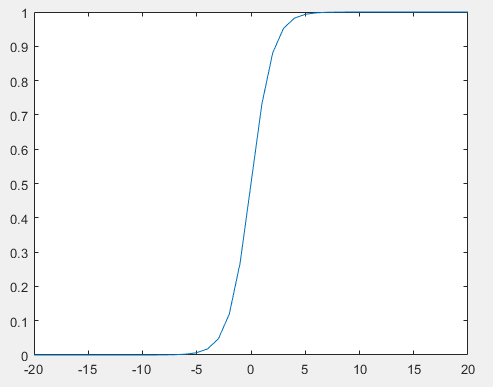如要将此曲线应用在步进电机的加、减速过程中，需要将方程在XY坐标系进行平移，同时对曲线进行拉升变化。对于初速度为𝑣s，末速度为𝑣e，运动步数为2n的纯加/减速段，对于其中第i步，可以规划速度为：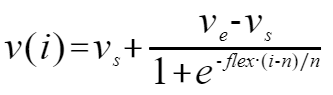（1-1）
其中flex参数是用来调节区间范围的，使用该方法规划的速度曲线关于中心点对称。比如当𝑣s=10，𝑣e=100,2n=2，flex=5时，规划的速度曲线如下图所示。由于关于中心点对称，所以S速度曲线(图中紫色曲线)包围的面积等价于从初速度𝑣s按恒定加速度加速到𝑣e的曲线（图中绿色曲线）所包围的面积。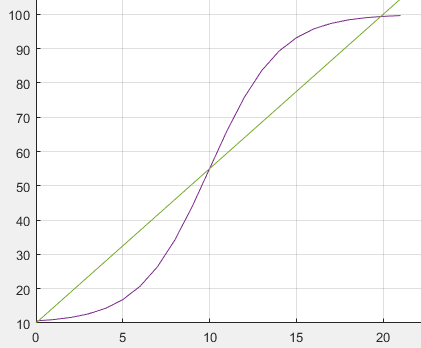所以可以先按梯形曲线规划速度曲线，然后对于其中的加速度段和减速度段，分别进行按照式（5-2）进行s速度曲线规划。则已知初速度𝑣s，末速度𝑣e，线段距离s，加速度a，进行s曲线速度规划步骤为：
进行梯形速度规划，算出加速段距离s1,加速和匀速段总距离s2，减速度段距离s3，以及最大加速度𝑣max对于加速段，按照式（1-1）方法执行s形速度规划对于减速段，按照式(1-1)方法执行s形速度规
流程示意图如下：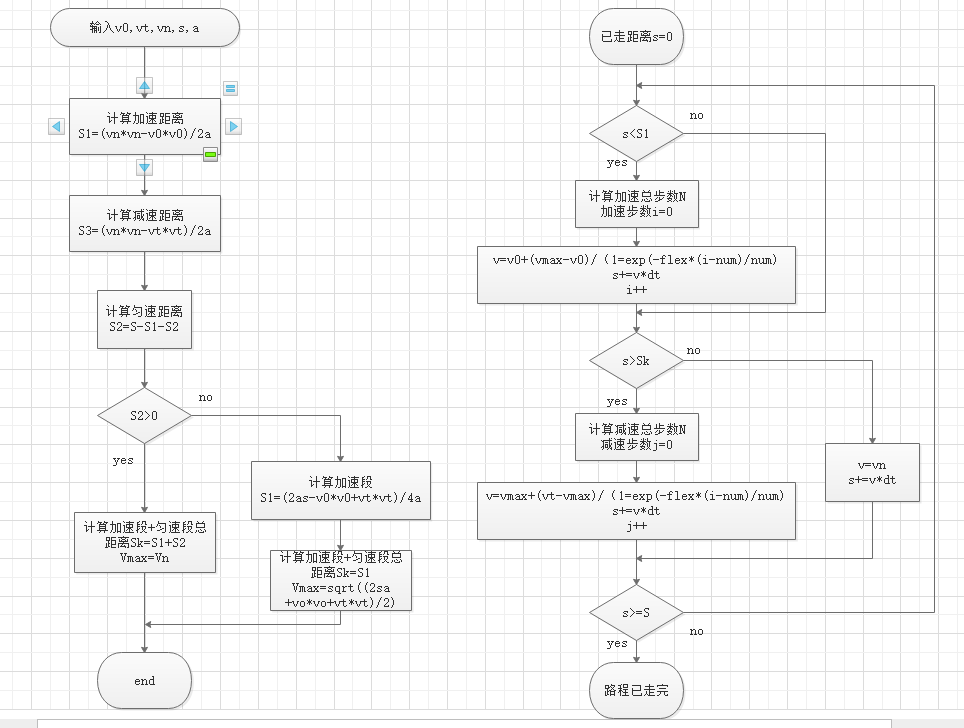展开全文• 7段S型曲线速度规划matlab实现,采用连续的，线性的加速度曲线，速度曲线抛物线过渡的线段组成。给定起始角度，最大速度、最大加速度、最大加加速度等参数，实现位置曲线，速度曲线，加速度曲线、加加速度曲线的规划...
• 文章目录1 无人驾驶的动作规划2 轨迹规划2.1 车辆模型2.2 道路定义2.3 候选轨迹生成2.4 根据轨迹点构建有向图并搜索3 速度规划3.1 S-T图算法3.2 设置Cost 1 无人驾驶的动作规划 动作规划的目的：是将上游行为决策...


文章目录
1 无人驾驶的动作规划2 轨迹规划2.1 车辆模型2.2 道路定义2.3 候选轨迹生成2.4 根据轨迹点构建有向图并搜索
3 速度规划3.1 S-T图算法3.2 设置Cost

1 无人驾驶的动作规划
动作规划的目的：是将上游行为决策（behavior）的宏观指令解释成一条带有时间信息的轨迹曲线（trajectory），最后给底层的反馈控制。
一种简单的思路是，将动作规划问题拆分成两个问题：
轨迹规划（Trajectory Planning）：二维平面上的优化轨迹问题。速度规划（Speed Planning）：选定轨迹后，用什么样的速度行驶这条轨迹。
分开优化不一定能保证总体最优解，但在实际工程实践中，分开优化是更实际有效的解决方案。

2 轨迹规划
2.1 车辆模型
车辆的姿态向量为

x

‾

=

(

x

,

y

,

θ

,

κ

,

υ

)

\overline{x}=(x,y,\theta,\kappa,\upsilon)

符号含义

(

x

,

y

)

(x,y)

车辆在二维片面内的位置

θ

\theta

车辆的朝向

κ

\kappa

曲率，即朝向

θ

\theta

的变化率

υ

\upsilon

车辆的速度，即轨迹任意点的切线速度
这些姿态变量满足以下关系：

x

˙

=

v

cos

⁡

θ

y

˙

=

v

sin

⁡

θ

θ

˙

=

v

κ

\begin{aligned} \dot{x} &amp;=v \cos \theta \\ \dot{y} &amp;=v \sin \theta \\ \dot{\theta} &amp;=v \kappa \end{aligned}

轨迹相对于车辆姿态的系统关系为：

d

x

/

d

s

=

cos

⁡

(

θ

(

s

)

)

d

y

/

d

s

=

sin

⁡

(

θ

(

s

)

)

d

θ

/

d

s

=

κ

(

s

)

\begin{array}{l}{\mathrm{d} x / \mathrm{d} s=\cos (\theta(s))} \\ {\mathrm{d} y / \mathrm{d} s=\sin (\theta(s))} \\ {\mathrm{d} \theta / \mathrm{d} s=\kappa(s)}\end{array}

2.2 道路定义
轨迹规划算法非常依赖于地图中对于道路中心线（Center Line）的定义，用一个采样函数去定义道路，采样函数为

r

(

s

)

=

[

r

x

(

s

)

,

r

y

(

s

)

,

r

θ

(

s

)

,

r

κ

(

s

)

]

r(s)=\left[r_{x}(s), r_{y}(s), r_{\theta}(s), r_{\kappa}(s)\right]

，车辆姿态点和道路坐标系以及道路采样函数的关系满足：

x

r

(

s

,

l

)

=

r

x

(

s

)

+

l

cos

⁡

(

r

θ

(

s

)

+

π

/

2

)

y

r

(

s

,

l

)

=

r

y

(

s

)

+

l

sin

⁡

(

r

θ

(

s

)

+

π

/

2

)

θ

r

(

s

,

l

)

=

r

θ

(

s

)

κ

r

(

s

,

l

)

=

(

r

κ

(

s

)

−

1

−

l

)

−

1

\begin{array}{l}{x_{r}(s, l)=r_{x}(s)+l \cos \left(r_{\theta}(s)+\pi / 2\right)} \\ {y_{r}(s, l)=r_{y}(s)+l \sin \left(r_{\theta}(s)+\pi / 2\right)} \\ {\theta_{r}(s, l)=r_{\theta}(s)} \\ {\kappa_{r}(s, l)=\left(r_{\kappa}(s)^{-1}-l\right)^{-1}}\end{array}

符号含义

s

s

纵向位移，道路中心线切线方向位移

l

l

横向位移
假设对于某条道路Lane(k)，纵向宽度

l

k

l_k

不变。那么该道路可以标示为一个随着中心线横向位移的点集

{

p

(

s

,

l

k

)

:

s

∈

R

+

}

\left\{p\left(s, l_{k}\right) : s \in \boldsymbol{R}^{+}\right\}

，这样的一个坐标系统为SL坐标系统。2.3 候选轨迹生成
首先，定义车辆的轨迹（Trajectory）为一个从[0,1]区间到车辆姿态向量集合

C

=

{

x

⃗

}

C=\{\vec{x}\}

的连续映射：

ρ

:

[

0

,

1

]

→

C

\rho :[0,1] \rightarrow C

，或者理解为一系列车辆姿态的序列。
符号含义

ρ

1

(

0

)

\rho_{1}(0)

第一条道路的起点姿态

ρ

1

(

1

)

\rho_{1}(1)

第一条道路的终点姿态

q

init

q_{\text {init}}

广义上的，初始姿态向量，

q

init

=

(

x

I

,

y

I

,

θ

l

,

κ

l

)

q_{\text { init }}=\left(x_{I}, y_{I}, \theta_{l}, \kappa_{l}\right)

q

e

n

d

q_{\mathrm{ end}}

广义上的，目标姿态向量，

q

g

o

a

l

=

(

x

G

,

y

G

,

θ

G

,

k

G

)

q_{\mathrm{goal}}=\left(x_{G}, y_{G}, \theta_{G}, k_{G}\right)

轨迹优化的目标，就是在所有可能轨迹中，筛选出满足边界条件的轨迹曲线，再寻找一条最平滑，Cost最低的曲线。候选轨迹曲线用类似路由寻径模块中的“撒点”采样生成，这样，一条轨迹就可以看成是沿着Lane的中心线纵向位移方向，连接不同Trajectory Point的平滑曲线。这里，采用多项式螺旋线连接轨迹点，生成候选曲线。多项式螺旋线，代表了一类曲率用弧长（对应这里的s）多项式函数来表示的曲线簇。一般使用三阶或者五阶多项式螺旋线，曲率

κ

\kappa

和轨迹弧长

s

s

的关系为：

三

阶

：

k

(

s

)

=

k

0

+

k

1

s

+

k

2

s

2

+

k

3

s

3

三阶：k(s)=k_{0}+k_{1} s+k_{2} s^{2}+k_{3} s^{3}

五

阶

：

k

(

s

)

=

k

0

+

k

1

s

+

k

2

s

2

+

k

3

s

3

+

k

4

s

4

+

k

5

s

5

五阶：k(s)=k_{0}+k_{1} s+k_{2} s^{2}+k_{3} s^{3}+k_{4} s^{4}+k_{5} s^{5}

三阶和五阶的区别为：三阶多项式会导致曲率的二阶导数

d

κ

2

/

d

s

2

\mathrm{d} \kappa^{2} / \mathrm{d} s^{2}

（对应方向盘转速）不连续，而五阶可以同事保持曲率的一阶导数和二阶导数的连续性。最后，最优利用梯度下降方法搜索，得到三阶（五阶）螺旋线连接的轨迹。
2.4 根据轨迹点构建有向图并搜索
在车辆模型，道路模型，轨迹点模型，和多项式螺旋曲线的设定下，轨迹规划问题可以简化为：

∣

l

total

/

Δ

l

∣

×

∣

s

total

/

Δ

s

∣

\left|l_{\text { total }} / \Delta l\right| \times\left|s_{\text { total }} / \Delta s\right|

个轨迹点连接成的

∣

l

total

/

Δ

l

∣

∣

s

ual

/

Δ

r

∣

\left|l_{\text { total }} / \Delta l\right|^{\left|s_{\text { ual }} / \Delta r\right|}

条候选曲线的搜索问题。
所有的轨迹点构成了一个图

G

=

(

V

,

E

)

G=(V, E)

。
符号含义

l

t

o

t

a

l

l_{total}

道路总宽度

Δ

l

\Delta l

宽度的最小采样长度

s

t

o

t

a

l

s_{total}

道路总长度

Δ

s

\Delta s

长度的最小采样长度

V

V

轨迹点，

v

∈

V

,

v

=

(

x

,

y

,

s

,

l

)

v \in V, v=(x, y, s, l)

E

E

三阶（五阶）多项式螺旋曲线

3 速度规划
3.1 S-T图算法
速度规划的任务，是在动作规划的轨迹上，考虑下游执行限制和行为决策结果，在每个轨迹点上，加入速度和加速度信息。
速度规划主要考虑对动态障碍物的规避，这里引入S-T（纵向位移 - 时间）的概念，并把无人车的速度规划问题，抽象到在S-T图上搜索的问题进行求解。
任何一个S-T图都基于一条已经给定的轨迹曲线，根据预测模块对动态障碍物的轨迹预测，每个动态障碍物都会在这条给定的轨迹上有所投影，从而产生一定S-T区域的覆盖，这里举一个S-T图速度优化算法的例子。图中，无人车正在思考轨迹规划要选取的换道轨迹，目标车道有前车b，后车a，则a和b在轨迹上的投影就是平行于s轴的线段，随着t的增加，这个投影平行四边形也会不断向右延伸。
模仿轨迹规划的地图划分，把地图分成小网格（Lattice Grid），对每个网格赋予Cost，最终速度规划问题就可以归纳为：从起点

(

s

=

0

,

t

=

0

)

(s=0,t=0)

到终点

(

s

=

S

e

n

d

,

t

&gt;

0

)

(s=S_{end},t&gt;0)

在网格上寻找最小路径Cost的搜索问题。
图中3种方案分别为：
方案说明Speed Plan 3加速直接超过a和bSpeed Plan 2超过a（Overtake），然后让b先过（Yield）Speed Plan 1让车a和b，一直跟在后面
3.2 设置Cost
根据决策信息，可以灵活调整障碍物周边的Cost，来达到速度调整的目的。例如，
上游决定对a超车，就在S-T图上将a运动轨迹上方网格的Cost调小； 上游决定对b让车，就在S-T图上将b运动轨迹下方网格的Cost调小； 为了避免碰撞，所有动态障碍物轨迹经过的网格Cost都需要调大。
因此，设置Cost是速度规划中S-T图算法的关键，在这个基础上，利用A*或者Dijkstra搜索算法，就能得到最小Cost轨迹。在得到了轨迹之后，通过计算任意轨迹点处的一阶导数和二阶倒数，就能计算出速度和加速度，从而完成速度规划的计算。

参考文章
《第一本无人驾驶技术书》刘少山
在Typora中输入公式
Typora开启行内公式
常用数学符号的 LaTeX 表示方法
一份不太简短的LATEX2ε介绍【精华】
展开全文无人驾驶 轨迹规划
• ## 梯形速度规划算法

千次阅读 2020-01-14 18:40:39
梯形速度是运动控制系统使用的最简单的一种速度规划方式，其速度曲线如下图所示： v0为初速度，vn为匀速运行速度，vt为末速度，加速和减速阶段加速度为a，总路程为S。 则加速阶段走过的路程S1=(vn*vn-v0*v0)/2a,...
梯形速度是运动控制系统使用的最简单的一种速度规划方式，其速度曲线如下图所示：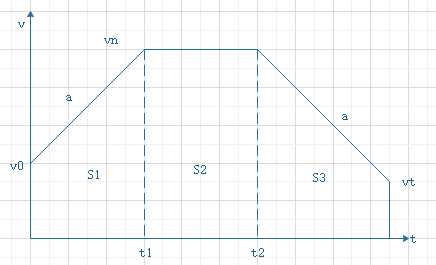v0为初速度，vn为匀速运行速度，vt为末速度，加速和减速阶段加速度为a，总路程为S。
则加速阶段走过的路程S1=(vn*vn-v0*v0)/2a,减速阶段走过的路程S3=(vn*vn-vt*vt)/2a，
则匀速阶段走过的路程S2=S-S1-S2
若S2<=0，代表此时没有匀速阶段，此时梯形速度退化成下图：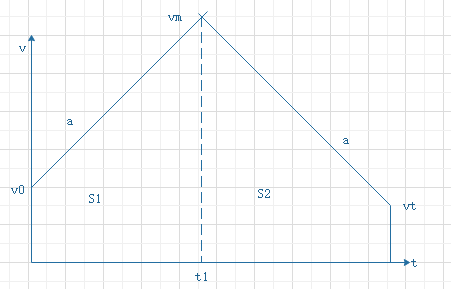加速段和减速段相交点位最大速度Vm，由下列关系式成立：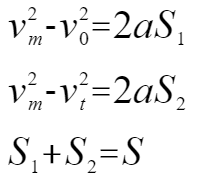则推导出：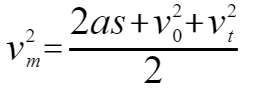带入算得：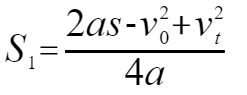（1-1）
S1为加速阶段走过的总路程，若S1<0，代表没有加速阶段只有减速阶段，若S2>S，代表只有加速阶段没有减速阶段。
最后总结一下梯形加速度规划方案：
在规划函数中：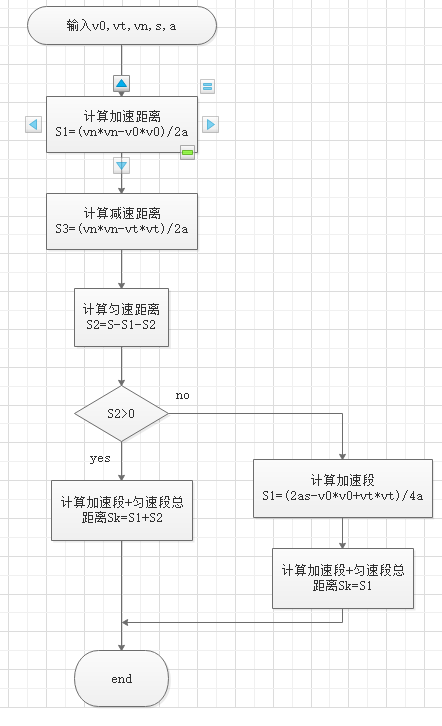在速度生成函数中：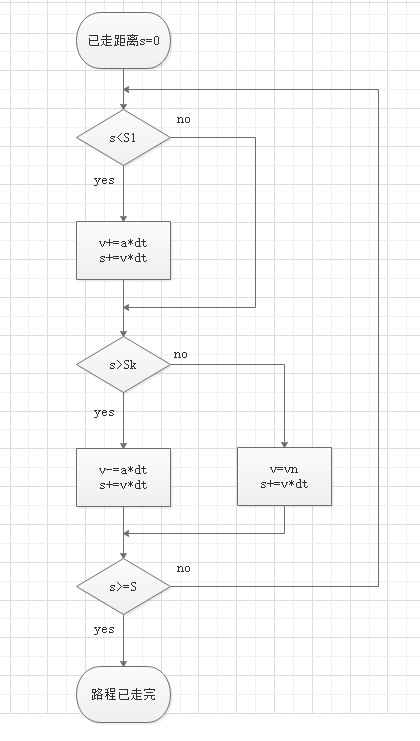展开全文• 一、双S型速度规划解决了梯形加速度不连续的问题 梯形加减速会产生不连续的加速度，从机械系统的角度来说，产生不必要的冲击力。这些现象不仅会损坏机械结构（如电机）还会产生不必要的振动。双S型速度规划的速度...
• 嵌入式数控插补系统速度规划研究及软件架构设计_余炼.caj
• 不使用bezier库；改进Bizuer，避免在重复输出控制点； 同时将等间隔的Bizuer曲线改进为等间距的Bizuer曲线，避免因为距离过短导致点过于密集；增加速度规划的功能
• 基于限速预测的一点式列车自动驾驶速度规划.pdf
• 为了实现数控系统连续轨迹的速度规划，本文提出了混合进给速度的规划方法。此方法通过建立转弯处加工路径和加工误差的数学模型，实现了转弯处进给速度的控制。实验结果表明，这种方法不仅可以极大的提高机床的加工...
• Apollo路径-速度规划轨迹生成方法 Apollo是百度开源的无人驾驶解决方案，其中规划中会按照不同的场景生成一条临时的路径，通过临时路径来障碍物规避，或者临时变道。 情况 假设前面有一个缓慢行驶的汽车离我们...Apollo 无人驾驶
• 针对一类严格反馈形式的非线性二阶多输入多输出系统，提出一种带有加速度规划的输出跟踪动态控制策略．引入一个代替时间变量的路径参数用以规划路径跟踪时的加速度，回避了设计内环加速度控制回路的常规方法，简化了...
• 基于B样条曲线理论，提出了3次B样条曲线插补算法，通过预判加工速度，采用3次B样条曲线不同段间连接点的切矢量求解，建立以时间为参数的样条曲线方程，同步完成插补轨迹规划和速度规划.经过仿真分析表明，该算法的...曲线插补
• 行业分类-化学冶金-一种基于强化学习的机器人速度规划方法和系统.zip
• 文章目录一、7种速度规划对比1、梯形速度规划2、余弦速度规划3、多项式速度规划4、7段S型速度规划5、7段修正S型速度规划6、15段S型速度规划7、31段S型速度规划二、7种速度规划示例演示 一、7种速度规划对比   一般...
• S-T Graph 是将有可能和path有overlap的障碍物投影到该path上面，生成st bounary。而速度规划则根据生成的st boundary进行纵向规划，其中s是指主车在该path上的行驶里程。自动驾驶 算法
• ## Matlab T型速度规划

千次阅读 2018-09-25 23:05:01
%% 梯形速度曲线 %% 假定初始速度和最终速度为零，给定最大的加速度、运行时间、开始角度 、结束角度 function trapezoid() %给定初始条件 t0=0; tf=10; q0=0; qf=20; a_max=1; if a_max&gt;= 4*(qf-q0)/(tf...
• 关节空间 VS 操作空间 　关节空间与操作空间轨迹规划流程图如下（上标$i$和$f$分别代表起始位置...可以根据机器人或驱动器手册直接确定最大速度或力矩 其缺点是对应操作空间的轨迹无法预测，增加了机械臂与...
• 首先，将障碍物的轨迹映射到s-t graph，随后计算出障碍物的轨迹（prior过程，障碍物在速度方面不存在decision，因此此次计算轨迹是withoutdecision），并将其放置boundaries集合中，随后设置速度最大限制（沿规划好...
• 主要世界绍集中运动控制的方法，以及各自的厂家，配了相应的图片运动控制
• 完整的AMD段包含以下三个阶段，分别是： 加速段 AP （Acceleration Phase） 匀速段 MP（Maximum Phase） 减速段 DP （Deceleration Phase） ...AD段存在，期望加速度与实际运行加速度均小于期望加速度； 仅A段
• 加加速度 j(t)={J0≤t≤t1−Jt1≤t≤t20t2≤t≤t3−Jt3≤t≤t4Jt4≤t≤t5 j(t)=\begin{cases} J & 0\leq t \leq t_1\\ -J & t_1\leq t \leq t_2\\ 0 & t_2\leq t \leq t_3\\ -J & t_3\leq t \leq ...算法
• 基于目标距离的S型速度曲线规划 ...算法计算由C语言实现 速度规划的数据写入到了mem_v.txt文档内， 可以使用excel将文档内的数据导入，（mem_v.txt内数据的分隔符为逗号‘,’）， 然后画折线图即可。详见帮助文档。...